# Normal Mapping without Precomputed Tangent Space Vectors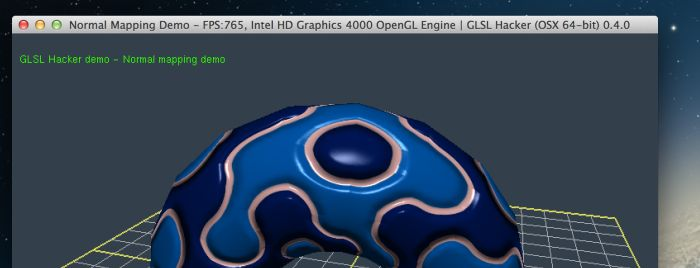What a nice opportunity to stick to the latest news of the graphics programming world. I just released the first version of GLSL Hacker and the support of precomputed tangent vectors is among the zillion of features that are missing in this first version. Tangent vectors (or TBN for Tangent Binormal Normal) are useful in many situations like bump/normal mapping and the TBN vectors are usually precomputed from texture coordinates and stored as a vertex attribute.

When precomputed tangent vectors are not present, I usually use the following method to compute them in the vertex shader:

```vec3 t;
vec3 b;
vec3 c1 = cross(vertex_normal, vec3(0.0, 0.0, 1.0));
vec3 c2 = cross(vertex_normal, vec3(0.0, 1.0, 0.0));
if (length(c1) > length(c2))
t = c1;
else
t = c2;
t = normalize(t);
b = normalize(cross(vertex_normal, t));
```

This method is fast but is approximative as you can show on the following picture: wee see a kind of pattern due to the imprecision of that method: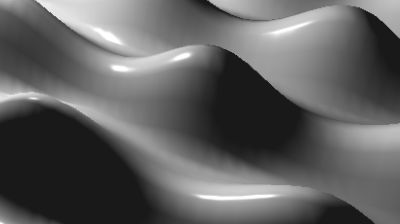The same method is also the source of the white line that appears on some tessellated objects like on the following picture: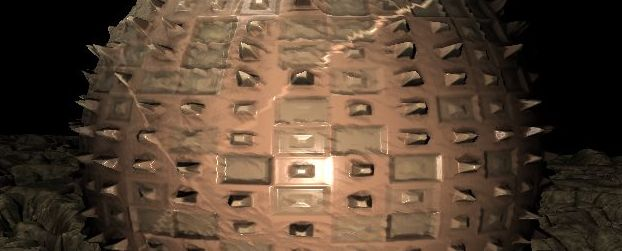MSI Kombustor, lighting glitch in tessellated object

Yesterday, I found in my tweets a link on this article: Followup: Normal Mapping Without Precomputed Tangents. The article explains in detail the math behind TBN space and near the end of the article, we discover the magic functions to compute in real time a perturbed normal vector from a normal map:
mat3 cotangent_frame(vec3 N, vec3 p, vec2 uv)
and
vec3 perturb_normal(vec3 N, vec3 V, vec2 texcoord).

Okay, let’s do a test in GLSL Hacker. I quickly coded a small demo that renders a normal-mapped torus using both previous functions. The GLSL code works fine under Windows (GTX 680 + R310.90), OSX 10.8 (GeForce GT 650M or Intel HD 4000) and Linux (Mint 13 + GTX 680 + R313.18):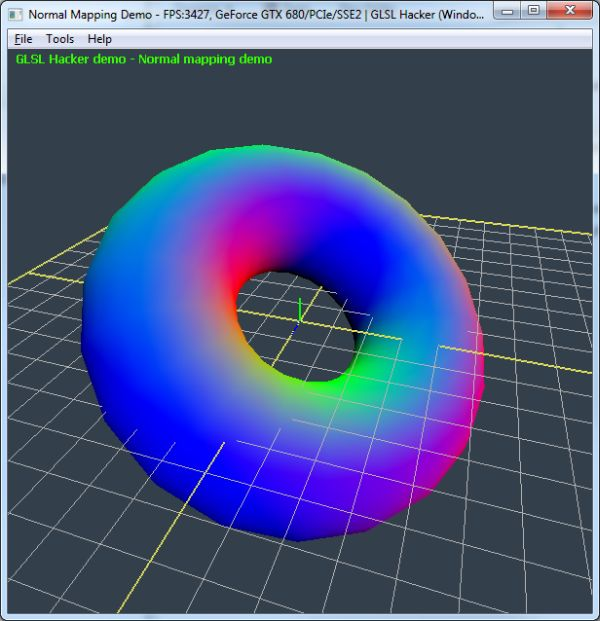Regular normal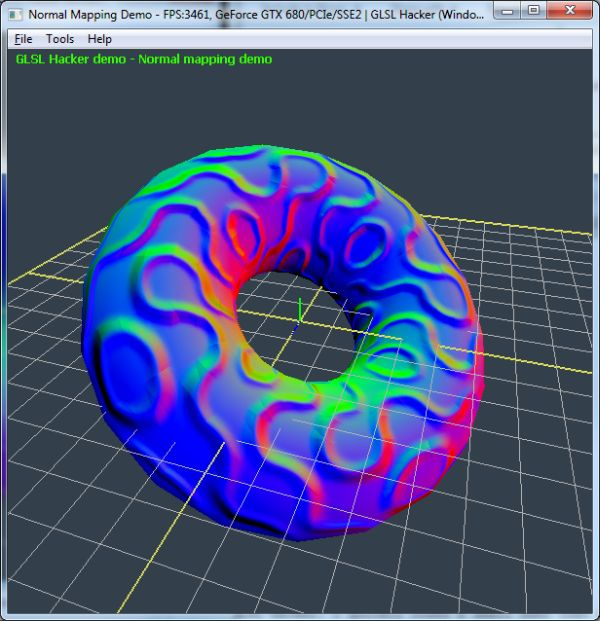Perturbed normal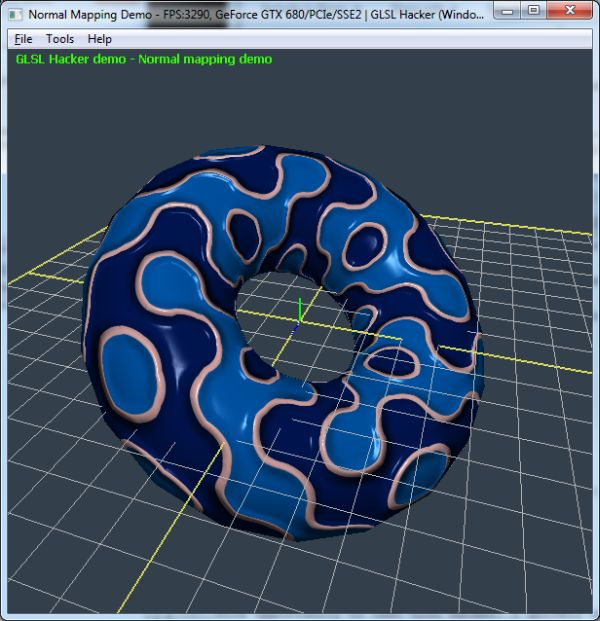Final result: normal mapping + Phong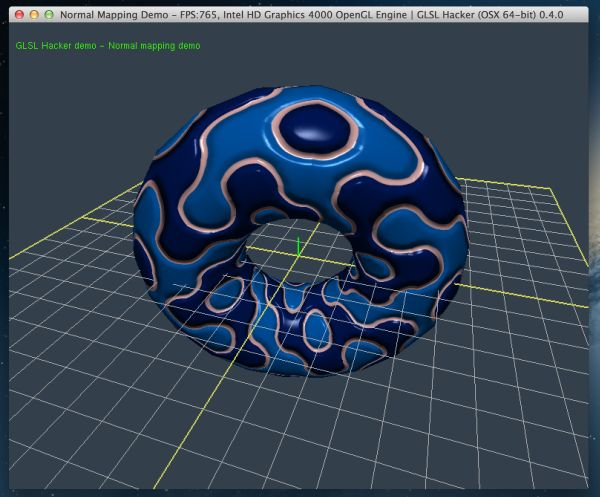normal mapping + Phong, OSX + Intel HD 4000 integrated GPU

The normal mapping works fine and the result is good. I think I won’t add precomputed tangent vector support in GLSL Hacker for the moment.

You can find the complete GLSL Hacker demo in the GLSL_Normal_Mapping/ folder of the Code Sample Pack. The demo is available in three versions: OpenGL 2.1, OpenGL 3.2 and OpenGL 4.2.

Here is the complete OpenGL 3.2+ GLSL program (vertex shader + pixel shader) that performs the normal mapping with Phong lighting:

```#version 150
in vec4 gxl3d_Position;
in vec4 gxl3d_Normal;
in vec4 gxl3d_TexCoord0;
out vec4 Vertex_UV;
out vec4 Vertex_Normal;
out vec4 Vertex_LightDir;
out vec4 Vertex_EyeVec;

// Automatically passed by GLSL Hacker
uniform mat4 gxl3d_ModelViewProjectionMatrix;

// Automatically passed by GLSL Hacker
uniform mat4 gxl3d_ModelViewMatrix;

uniform vec4 light_position;
uniform vec4 uv_tiling;

void main()
{
gl_Position = gxl3d_ModelViewProjectionMatrix * gxl3d_Position;
Vertex_UV = gxl3d_TexCoord0 * uv_tiling;
Vertex_Normal = gxl3d_ModelViewMatrix  * gxl3d_Normal;
vec4 view_vertex = gxl3d_ModelViewMatrix * gxl3d_Position;
Vertex_LightDir = light_position - view_vertex;
Vertex_EyeVec = -view_vertex;
}
```

```#version 150
precision highp float;
uniform sampler2D tex0; // color map
uniform sampler2D tex1; // normal map
uniform vec4 light_diffuse;
uniform vec4 material_diffuse;
uniform vec4 light_specular;
uniform vec4 material_specular;
uniform float material_shininess;
in vec4 Vertex_UV;
in vec4 Vertex_Normal;
in vec4 Vertex_LightDir;
in vec4 Vertex_EyeVec;
out vec4 Out_Color;

// http://www.thetenthplanet.de/archives/1180
mat3 cotangent_frame(vec3 N, vec3 p, vec2 uv)
{
// get edge vectors of the pixel triangle
vec3 dp1 = dFdx( p );
vec3 dp2 = dFdy( p );
vec2 duv1 = dFdx( uv );
vec2 duv2 = dFdy( uv );

// solve the linear system
vec3 dp2perp = cross( dp2, N );
vec3 dp1perp = cross( N, dp1 );
vec3 T = dp2perp * duv1.x + dp1perp * duv2.x;
vec3 B = dp2perp * duv1.y + dp1perp * duv2.y;

// construct a scale-invariant frame
float invmax = inversesqrt( max( dot(T,T), dot(B,B) ) );
return mat3( T * invmax, B * invmax, N );
}

vec3 perturb_normal( vec3 N, vec3 V, vec2 texcoord )
{
// assume N, the interpolated vertex normal and
// V, the view vector (vertex to eye)
vec3 map = texture(tex1, texcoord ).xyz;
map = map * 255./127. - 128./127.;
mat3 TBN = cotangent_frame(N, -V, texcoord);
return normalize(TBN * map);
}

void main()
{
vec2 uv = Vertex_UV.xy;

vec3 N = normalize(Vertex_Normal.xyz);
vec3 L = normalize(Vertex_LightDir.xyz);
vec3 V = normalize(Vertex_EyeVec.xyz);
vec3 PN = perturb_normal(N, V, uv);

vec4 tex01_color = texture(tex0, uv).rgba;
vec4 final_color = vec4(0.2, 0.15, 0.15, 1.0) * tex01_color;

float lambertTerm = dot(PN, L);
if (lambertTerm > 0.0)
{
final_color += light_diffuse * material_diffuse * lambertTerm * tex01_color;

vec3 E = normalize(Vertex_EyeVec.xyz);
vec3 R = reflect(-L, PN);
float specular = pow( max(dot(R, E), 0.0), material_shininess);
final_color += light_specular * material_specular * specular;
}
Out_Color.rgb = final_color.rgb;
//Out_Color.rgb = PN.rgb;
//Out_Color.rgb = N.rgb;
Out_Color.a = 1.0;
}
```

## 3 thoughts on “Normal Mapping without Precomputed Tangent Space Vectors”

1.Ming

As I remember dFdx() and dFdy() is approximated with a 2×2 block of pixels in most GPU.

Is there any blocky artifacts when the camera is zoomed very close to the object’s surface?

2.xernobyl

Some error and performance analysis would be appreciated 🙂

3.Sam

Note; for some reason, this method produces flipped normals on the Intel HD3000.